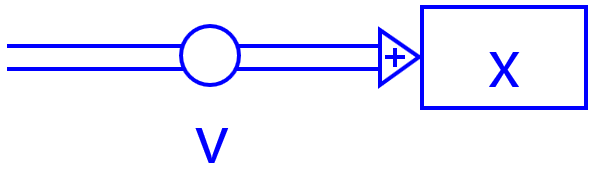# 4. Overview of models and model equations

## 4.1 Force and motion

Modelling is introduced in many textbooks in the subject of Force and Motion.1 Students are familiar with motion and its graphical representations through lessons on kinematics. This allows them to interpret and assess graphs that are the outcomes of modelling tasks. In addition, quantities such as velocity and acceleration indicate a change; that makes it easier for students to accept the iterative steps in the calculations. The main reason, however, is that the iterative method can deal with dynamic problems that cannot be solved by hand at secondary school level.

Below is an overview of some dynamic models in the subdomain Force and Motion in the physics syllabus on Motion and Interaction,2,3 with corresponding files for the Coach 7 modelling environment.4

1. This model forms the basis of the step-by-step calculation of movements, originally conceived by Isaac Newton. The calculation rule for uniform motion is that the new value for the position is given by the previous value plus the change in the time step $$\Delta t$$.

Formula in Binas:

$$v = dx/dt$$

Difference equation:

$$\Delta x = v \cdot \Delta t$$

Text-based model

$$\begin{array}{l} x = x + v \cdot dt\\ t = t + dt \end{array}$$

Graphical model